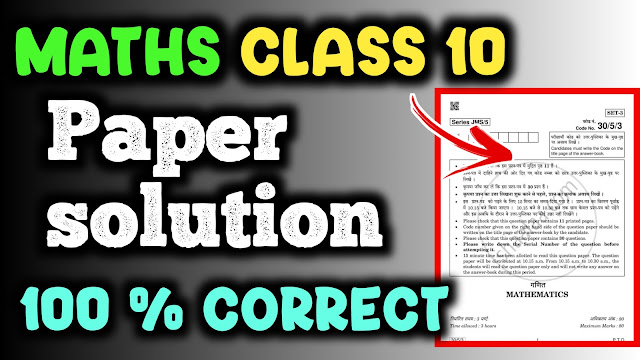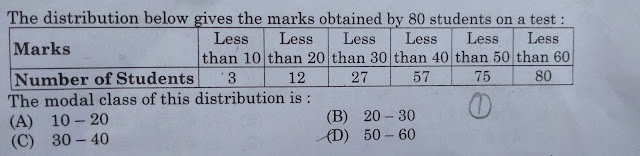# Math class 10 Answer key 2023

Hello students, here the solution of Class 10 math paper is given below.Class 10 math paper solution 2023

*Note:- some answers are not given because we are solving them and will be updated in some time*

### Class 10 math paper solution 2023

Below the answers of class 10 Math paper solution is given.

#### 1. In what ratio, does the x-axis divide the line segment joining the points A(3,6) and B(-12,-3)?

y=m1y2+m2y1/m1+m2
= K×-3+1×6/k+1=0
= -3k=-6
= k=-6/-3
K=2/1

2:1

And:- 47.5°

#### 3. If 2tanA=3, then the value of 4sinA+3cosA/4sinA-3cosA is

And:- 3

=4×3/√3 + 3×2/√3/4×3/√3 - 3×2/√3
= 18/√3/6/√3
=18/√3×√3/6
=3

#### 5. The distribution below gives the marks obtained by 80 students on a test:Class 10 cbse board maths paper solution 2023

#### 19. Assertion (A) : The perimeter of Triangle ABC is a rational number.Reason(R): The sum of the squares of two rational numbers is always rational.

Answer:- Both assertion (A) and Reason (R) are true and Reason (R) is the correct explanation of assertion (A)

#### 20. Assertion (A) : Point P(0,2) is the point of intersection of y-axis with the line 3x+2y=4.Reason(R): The distance of point P(0,2) from x-axis is 2 units.

Answer:- Assertion (A) is false but Reason (R) is true.

#### 23. (A) solve the pair of Equations x=5 and y=7 graphically.(B) using graphically method, find whether pair of equations x=0 and y= -3 is consistent or not.

Answer:- However, the equation x = 5 represents a vertical line passing through the point (5,0) on the x-axis. The equation y = 7 represents a horizontal line passing through the point (0,7) on the y-axis. These lines do not intersect, as they are parallel to each other.

Therefore, there is no solution to this pair of linear equations.

#### 27. (a) Two tangents TP and TQ are drawn to circle with centre O from an external point T. Prove that angle PTQ= 2 Angle OPQ.

Let the tangents TP and TQ intersect at point X, and let the radius of the circle be r. Since OP and OQ are radii of the circle, we have OP = OQ = r.

Since TP and TQ are tangents to the circle, we have:

∠OTP = ∠OTQ = 90° (tangents are perpendicular to the radius at the point of contact)

Now, we can apply the angle sum property of triangle TPX to obtain:

∠PTX + ∠PTQ + ∠XTQ = 180° (sum of angles in a triangle)

Since TP = TQ (both are tangents from point T), we have ∠PTX = ∠QTX, so:

2∠PTQ + ∠XTQ = 180°

Also, we know that ∠OTP = ∠OTQ, so:

∠OPQ = 1/2 (∠OTP + ∠OTQ) = 1/2 (2∠OTP) = ∠OTP

Therefore, we have:

∠XTQ = 2∠OPQ

Substituting this into the earlier equation, we get:

2∠PTQ + 2∠OPQ = 180°

Dividing both sides by 2, we obtain:

∠PTQ + ∠OPQ = 90°

Now, since ∠PTQ and ∠OPQ are both angles in triangle TPQ, we have:

∠PTQ + ∠OPQ + ∠PQT = 180°

Substituting ∠PTQ + ∠OPQ = 90°, we get:

90° + ∠PQT = 180°

Simplifying gives:

∠PQT = 90°

Therefore, in triangle OPQ, we have:

∠OPQ + ∠PQO + ∠OQP = 180° (sum of angles in a triangle)

Substituting ∠OPQ = ∠PTQ/2 and ∠PQT = 90°, we get:

∠PTQ/2 + ∠PQO + ∠OQP = 180°

Simplifying and rearranging gives:

∠PTQ/2 = ∠PQO + ∠OQP

Therefore, we have:

∠PTQ/2 = 2∠OPQ

Or equivalently,

∠PTQ = 2∠OPQ

Hence, we have proved that angle PTQ is twice angle OPQ.

#### (B) In the given figure, a circle is inscribed in a quadrilateral ABCD in which angle B= 90°. If AD = 17 cm, AB = 20 cm and DS 3 cm, then find the radius of the circle.

AP + BS = BQ + CR (Sum of opposite sides of a cyclic quadrilateral)

Substituting AP = AS = x, BS = BP = y, and CR = CQ = z, we get:

x + y = z + DS ...(1)

Also, since triangle ABP is a right triangle, we have:

AP^2 + BP^2 = AB^2

Substituting AP = x, BP = y, and AB = 20 cm, we get:

x^2 + y^2 = 400 ...(2)

Similarly, since triangle CQR is a right triangle, we have:

CQ^2 + QR^2 = CR^2

Substituting CQ = z, QR = r, and CR = z + r, we get:

z^2 + r^2 = (z + r)^2

Simplifying and rearranging, we get:

r = (z^2)/(2z + 2DS) ...(3)

Now, we need to find x, y, and z in terms of the given quantities. From equation (1), we have:

x + y - DS = z

Adding and subtracting equation (2), we get:

2x = 400 - 2y, and

2y = 400 - 2x

Substituting these into the above equation, we get:

2x - DS = 400 - 2x

Simplifying and solving for x, we obtain:

x = (400 - DS)/4

Similarly, we can solve for y:

y = (400 - DS)/4

Substituting these values of x and y into equation (1), we get:

(400 - DS)/2 = z

Substituting this into equation (3), we obtain:

r = [(400 - DS)^2]/[8(400 - DS)] = (400 - DS)/8 = 3/8

Therefore, the radius of the inscribed circle is 3/8 cm.

#### 28. Half of the difference between two numbers is 2. The sum of the greater number and twice the smaller number is 13. Find the numbers.

Answer:- Let the two numbers be x and y, where x is the greater number and y is the smaller number.

From the first condition, we have:

(x - y)/2 = 2

Multiplying both sides by 2, we get:

x - y = 4

From the second condition, we have:

x + 2y = 13

Now we have two equations with two unknowns:

x - y = 4 ...(1) x + 2y = 13 ...(2)

We can solve for x and y by eliminating one variable. Multiplying equation (1) by 2, we get:

2x - 2y = 8

Adding this equation to equation (2), we get:

3x = 21

Dividing both sides by 3, we get:

x = 7

Substituting this value of x into equation (1), we get:

7 - y = 4

Solving for y, we get:

y = 3

Therefore, the two numbers are 7 and 3.

#### 29. A room is in the form of cylinder surmounted by a hemi-spherical dome. The base radius of hemisphere is one-half the height of cylindrical part. Find total height of the room if it contains [ 1408/21] m3 of air. ( Take π= 22/7)

Let the height of the cylindrical part be 'h' and the base radius of the hemisphere be 'r'. Then, the total height of the room will be:

H = h + 2r ...(1)

The volume of the room can be calculated as the sum of the volume of the cylindrical part and half the volume of the hemispherical part, which is given as:

V = (1/2)πr^2h + (2/3)πr^3 ...(2)

Substituting the value of r in terms of h from the given condition, we get:

r = h/2

Substituting this value of r in equation (2), we get:

V = (1/2)π(h^2/4)h + (2/3)π(h^3/8)

Simplifying, we get:

V = πh^3/12 + πh^3/12

V = πh^3/6

Equating this to the given volume, we get:

πh^3/6 = 1408/21

Solving for h, we get:

h^3 = (1408/21) x 6/π

h^3 = 128

h = 4

Substituting this value of h in equation (1), we get:

H = h + 2r

H = 4 + 2(2)

H = 8

Therefore, the total height of the room is 8 m.

#### (b) An empty cone is of radius 3 cm and height 12 cm. Ice-cream is filled in it so that lower part of the cone which is 1/6 of the volume of  the cone is unfilled but hemisphere is formed on the top. Find volume of the ice-cream. (Take л= 3.14)

Answer:- The volume of the empty cone can be calculated using the formula:

Volume of cone = (1/3)πr^2h

Substituting the given values, we get:

Volume of cone = (1/3) x 3.14 x 3^2 x 12

Volume of cone = 113.04 cm^3

The lower part of the cone which is unfilled is 1/6 of the volume of the cone, so the volume of the unfilled part is:

Volume of unfilled = (1/6) x Vcone

Volume of unfilled = (1/6) x 113.04

Volume of unfilled = 18.84 cm^3

The volume of the ice-cream can be calculated as the difference between the volume of the hemisphere and the volume of the unfilled part of the cone. The radius of the hemisphere can be calculated as the radius of the cone, which is 3 cm. The volume of the hemisphere can be calculated using the formula:

Volume of hemisphere = (2/3)πr^3

Substituting the value of r, we get:

Volume of hemisphere = (2/3) x 3.14 x 3^3

Volume of hemisphere = 56.52 cm^3

Therefore, the volume of the ice-cream is:

Volume of ice-cream = Volume of hemisphere - Volume of unfilled

Volume of ice-cream = 56.52 - 18.84

Volume of ice-cream = 37.68 cm^3

Hence, the volume of the ice-cream is 37.68 cm^3.

#### 30. Prove that √5 is irrational number.

Answer:- To prove that √5 is an irrational number, we need to assume the opposite, i.e., we assume that √5 is a rational number. By definition, a rational number can be expressed as the ratio of two integers, say p and q, where q is not equal to zero and p and q have no common factors other than 1.

Assuming that √5 is rational, we can write:

√5 = p/q

Squaring both sides, we get:

5 = p^2/q^2

Multiplying both sides by q^2, we get:

5q^2 = p^2

This means that p^2 is divisible by 5, which implies that p must be a multiple of 5. Let p = 5k, where k is an integer. Substituting this value in the above equation, we get:

5q^2 = (5k)^2

5q^2 = 25k^2

Dividing both sides by 5, we get:

q^2 = 5k^2

This means that q^2 is divisible by 5, which implies that q must also be a multiple of 5.

However, we had assumed that p and q have no common factors other than 1, which contradicts the fact that p and q are both multiples of 5. Therefore, our assumption that √5 is a rational number leads to a contradiction, and we can conclude that √5 is an irrational number.